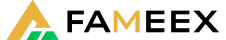Register
Support Center

FAMEEX provides a user-friendly, secure, reliable, and excellent crypto trading experience. Download our mobile APP and Trade your Bitcoin anywhere and whenever you want!To trade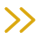Search
Support CenterOperation guideHow to Calculate your PnL
2022/03/04 20:23:17

If you would like to know more details, please read the whole article below.

Here is our tutorial video: https://www.youtube.com/watch?v=7t_YnXn3ioA

U-Futures Trading is an independent futures wallet for each user to trade. This Futures Wallet will be used to calculate your margin and balance.

1. Specifications of Futures Wallet2. Specifications of Positions3. How to Calculate your PnL?

Realized Profit and Loss is the revenue or loss that users earn after close positions.

(1) Realized PnL (after close position)

If you open only one direction of long/short positions:

Realized PnL = sum{The Amount of Close Position X (Close Price - Avg. Open Price)}

For example, a user opens a long position in the amount of 2 BTC at the price of 500 USDT, and then closes in the amount of 1 BTC at the price of 1,000 USDT.

The Realized PnL = 1 X (1,000-500) = 500 USDT

If you close one direction of long/short positions:

Realized PnL = sum{The Amount of Close Position X (Avg. Open Price - Close Price)}

For example, a user closes  a short position in the amount of 10 BTC at the price of 500 USDT, and then opens in the amount of 8 BTC at the price of 1,000 USDT.

The Realized PnL = 8 X (500-1,000) = -4,000 USDT

(2) Unrealized PnL (Positions size PnL)

Long Position of Unrealized PnL = (The Last Mark Price - Avg. Open Price) X Position Size

Short Position of Unrealized PnL = (Avg. Open Price - The Last Mark Price) X Position Size

4. Average Price

Average Price = (Position Amount X Avg. Price + New Position Amount X Avg. Open Price) / (Petition Amount + New Position Amount)

New Avg. Open Price = (Filled Position 1 X Filled Price 1 + Filled Position 2 X Filled Price 2 + …) / New Position Amount

New Position Amount = Open Position Amount of Filled Price 1 + Open Position Amount of Filled Price 2 + ...

For example, the last mark price is 600 USDT. A user holds 6 BTC with a long position at the avg. open price of 500 USDT, then buy 5 BTC of long position.

5. BTC of filled price as following:The 5 BTC Avg. Filled Price = (1 X 580 + 1 X 570 + 3 X 560) / 5 = 566 USDT

New Avg. Position Price = (6 X 500 + 5 X 566) / (6 + 5) = 530 USDT

New Position Amount = 5 + 6 = 11 BTC

5. Estimated Liquidation Price

(1) Estimated Liquidation Price of Cross Margin

For example, the formula for the Estimated Liquidation Price (ELP) of Cross Mode

= [Account Balance - Isolated Margin + (ELP - Average Position Price) X Size of Long Position + (Average Position Price - ELP) X Size of Short Position + Unrealized PnL - Maintenance Margin of Cross Contract ] / (Size of Long Position X ELP + Size of Short Position X ELP ) = Maintenance Margin Rate

(2) Estimated Liquidation Price of Isolated Margin

For example, the formula for the Estimated Liquidation Price (ELP) of Isolated Mode

=[ Isolated Margin + (ELP - Average Position Price) X Size of Long Position + (Average Position Price - ELP) X Size of Short Position] /  (Size of Long Position X ELP + Size of Short Position X ELP) = Maintenance Margin Rate

6. Bankruptcy Price

Traders need to keep a certain percentage of its fund as a maintenance margin. If user's margin is lower than the maintenance margin, it will trigger the auto deleveraging system or liquidation. Our system will close your positions at bankruptcy price. The actual filled price will depend on the last trade price.

(1) Bankruptcy Price of Cross Mode (BPC)

Bankruptcy Price of Cross Mode = Account Balance - Isolated Margin + Unrealized PnL - Maintenance Margin of Cross Contract +(BPC - Avg. Open Price) X Size of Long Position + (Avg. Open Price - BPC) X Size of Short Position - Size of Long Position X BPC X Transaction Fee Rate of Taker - Short of Position X BPC X Transaction Fee Rate of Taker = 0

(2) Bankruptcy Price of Isolated Mode (BPI)

Bankruptcy Price of Isolated Mode = Isolated Margin + (BPI - Avg. Open Price) X Size of Long Position + (Avg. Open Price - BPI) X Size of Short Position - Size of Long Position X BPC X Transaction Fee Rate of Taker - Short of Position X BPI X Transaction Fee Rate of Taker = 0

7. Return on Equity Rate

ROE% of Cross Margin = Unrealized PnL / Cost of Margin X 100%

ROE% of Isolated Margin = Unrealized PnL / (Mark Price X Position / Leverage) X 100%

•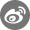•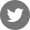•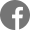•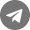•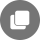Bugs Report:Safe@mail.fameex.info